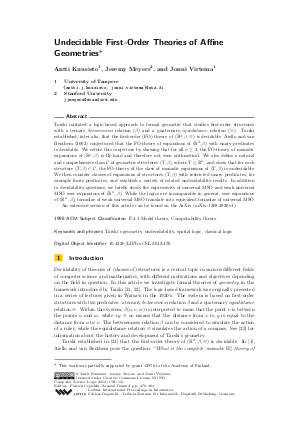Document# Undecidable First-Order Theories of Affine Geometries

### Authors Antti Kuusisto, Jeremy Meyers, Jonni Virtema## File

LIPIcs.CSL.2012.470.pdf
• Filesize: 0.49 MB
• 15 pages

## Cite As

Antti Kuusisto, Jeremy Meyers, and Jonni Virtema. Undecidable First-Order Theories of Affine Geometries. In Computer Science Logic (CSL'12) - 26th International Workshop/21st Annual Conference of the EACSL. Leibniz International Proceedings in Informatics (LIPIcs), Volume 16, pp. 470-484, Schloss Dagstuhl - Leibniz-Zentrum für Informatik (2012)
https://doi.org/10.4230/LIPIcs.CSL.2012.470

## Abstract

Tarski initiated a logic-based approach to formal geometry that studies first-order structures with a ternary betweenness relation (\beta) and a quaternary equidistance relation (\equiv). Tarski established, inter alia, that the first-order (FO) theory of (R^2,\beta,\equiv) is decidable. Aiello and van Benthem (2002) conjectured that the FO-theory of expansions of (R^2,\beta) with unary predicates is decidable. We refute this conjecture by showing that for all n > 1, the FO-theory of monadic expansions of (R^n,\beta) is Pi^1_1-hard and therefore not even arithmetical. We also define a natural and comprehensive class C of geometric structures (T,\beta), where T is a subset of R^n, and show that for each structure (T,\beta) in C, the FO-theory of the class of monadic expansions of (T,\beta) is undecidable. We then consider classes of expansions of structures (T,\beta) with restricted unary predicates, for example finite predicates, and establish a variety of related undecidability results. In addition to decidability questions, we briefly study the expressivity of universal MSO and weak universal MSO over expansions of (R^n,\beta). While the logics are incomparable in general, over expansions of (R^n,\beta), formulae of weak universal MSO translate into equivalent formulae of universal MSO. An extended version of this article can be found on the ArXiv (arXiv:1208.4930v1).
##### Keywords
• Tarski’s geometry
• undecidability
• spatial logic
• classical logic

## Metrics

• Access Statistics
• Total Accesses (updated on a weekly basis)
0